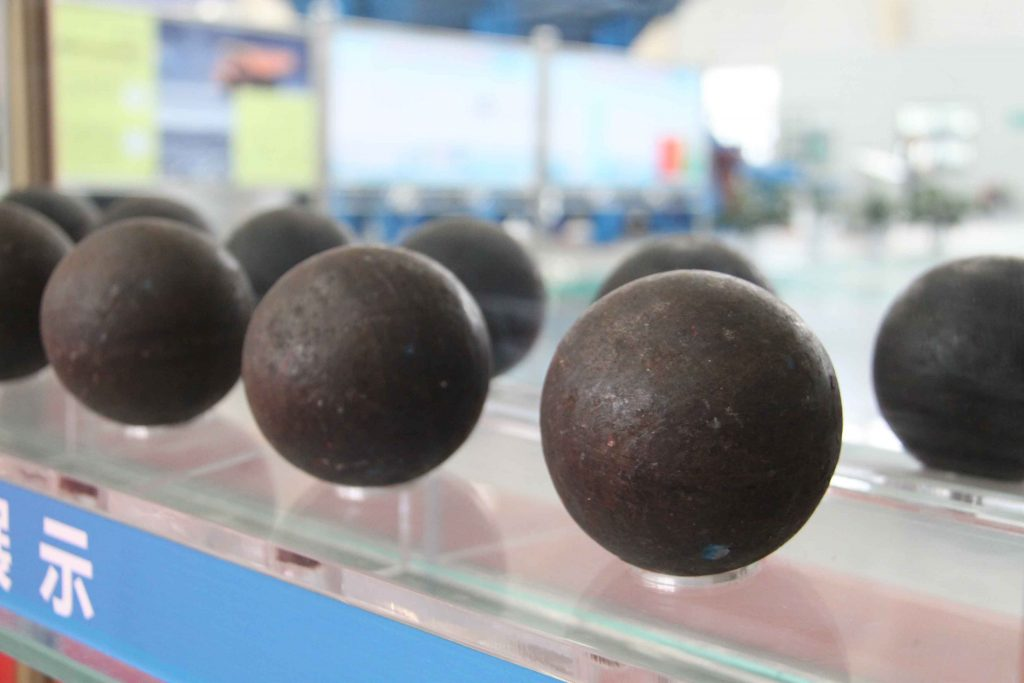# The formula for calculating the weight of grinding mill steel balls

Regarding the calculation of the weight of grinding mill steel balls, there are two different calculations due to the difference in steel ball density: one for forged steel balls and another for cast steel balls.hot rolled steel grinding ball

The density of forged steel balls is generally taken as 7.85, while cast steel balls are taken as 7.4. Forged steel has a higher density than cast iron, so it is important not to use a single formula for both:

1. Formula for calculating the weight of forged steel balls: Diameter (mm) × Diameter (mm) × Diameter (mm) × 4.1 / 1000000 = kg. This formula gives the weight of each corresponding diameter steel ball.
2. Formula for calculating the weight of cast steel balls: Diameter (mm) × Diameter (mm) × Diameter (mm) × 3.865 / 1000000 = kg. This formula gives the weight of each corresponding diameter steel ball.

For example:

(1) Forged steel ball with a diameter of 100mm: Formula: Diameter (mm) × Diameter (mm) × Diameter (mm) × 4.1 / 1000000 = kg Calculation: 100 × 100 × 100 × 4.1 / 1000000 = 4.1kg

(2) Cast steel ball with a diameter of 100mm: Formula: Diameter (mm) × Diameter (mm) × Diameter (mm) × 3.865 / 1000000 = kg Calculation: 100 × 100 × 100 × 3.865 / 1000000 = 3.865kg

If you want to trace the origin of these formulas, I can tell you that it is derived from a middle school math problem:

The formula for calculating the weight of a sphere = volume of the sphere × density = 4/3π(L/2) × (L/2) × (L/2) × density

For example, for a forged steel ball with a diameter D = 100mm = 10cm, we have R = 5cm and density M = 7.85g/cm3. Therefore, the weight of the steel ball G = 4/3π(L/2) × (L/2) × (L/2) × density = 4/3 × 3.1415926 × 5 × 5 × 5 × 7.85 = 4.09454236kg.

You may wonder why this number is different from the result obtained from the previous formula. The reason is simple: it is due to the approximation of pi and the assumed density of the steel ball. Pi is an infinite decimal, and the density of steel balls is usually taken as 7.85, but in reality, there are various alloy steels with different densities. The density also varies slightly depending on the forging process, with higher alloy content and forging processes resulting in slightly higher densities. That’s all there is to it.

You can also refer to the following diagram for a comparison of diameter and weight (forged steel balls):

 Ball diameter (mm) Ball volume (cm³/pc） Ball surface area (cm²/pc) Ball weight/pc （KG) Number of balls per ton（Pcs） Surface area of balls per ton（㎡） The weight of a cubic meter ball(ton) 20 4.2 12.6 0.033 30303 37.8 30 14.1 28.3 0.111 9000 25.4 4.85 35 22.4 38.5 0.176 5682 21.9 4.85 40 33.5 50.3 0.263 3800 19.1 4.76 50 65.5 78.5 0.614 1945 15.3 4.76 60 113 113 0.887 1127 12.7 4.66 70 180 154 1.413 708 10.9 75 220.8 176.6 1.733 577 10.19 4.6 80 268 201 2.1 476 9.57 4.6 90 382 231 3 333 8.46 100 524 314 4.13 243 7.63 4.56 110 697 380 5.47 183 6.95 120 905 452 7.104 141 6.37 125 1022 491 8.02 125 6.14 4.52 140 1436 616 11.273 89 5.48 Remarks：The specific gravity of the steel ball is calculated as 7.85/m³.
0 replies